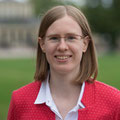# Research Group of Prof. Dr. Barbara Verfürth### Contact Information

Institut für Numerische Simulation
Friedrich-Hirzebruch-Allee 7
53115 Bonn
Phone: +49 228 73-69833
Office: FHA7 3.030
E-Mail: ed tod nnob-inu tod sni ta htreufreva tod b@foo tod de

## Research interests

• numerical methods for PDEs
• multiscale (finite element) methods
• numerical homogenization
• (time-harmonic) wave propagation, Helmholtz and Maxwell equations
• quasilinear PDEs

### Publications

#### Preprints

1. Fully discrete Heterogeneous Multiscale Method for parabolic problems with multiple spatial and temporal scales. D. Eckhardt and B. Verfürth. arXiv preprint 2210.04536, 2022.
2. Higher-order finite element methods for the nonlinear Helmholtz equation. B. Verfürth. arXiv preprint 2208.11027, 2022.

#### Articles

1. Modeling four-dimensional metamaterials: a T-matrix approach to describe time-varying metasurfaces. P. Garg, A. G. Lamprianidis, D. Beutel, T. Karamanos, B. Verfürth, and C. Rockstuhl. Opt. Express, 30(25):45832–45847, 2022.
2. Numerical Upscaling for Wave Equations with Time-Dependent Multiscale Coefficients. B. Maier and B. Verfürth. Multiscale Model. Simul., 20(4):1169–1190, 2022.
3. Multiscale scattering in nonlinear Kerr-type media. R. Maier and B. Verfürth. Math. Comp., 91(336):1655–1685, 2022.
4. Nonlinear Helmholtz equations with sign-changing diffusion coefficient. R. Mandel, Z. Moitier, and B. Verfürth. C. R. Math. Acad. Sci. Paris, 360:513–538, 2022.
5. An offline-online strategy for multiscale problems with random defects. A. Målqvist and B. Verfürth. ESAIM Math. Model. Numer. Anal., 56(1):237–260, 2022.
6. Numerical homogenization for nonlinear strongly monotone problems. B. Verfürth. IMA J. Numer. Anal., 42(2):1313–1338, 2022.
7. A multiscale method for heterogeneous bulk-surface coupling. R. Altmann and B. Verfürth. Multiscale Model. Simul., 19(1):374–400, 2021.
8. A generalized finite element method for problems with sign-changing coefficients. T. Chaumont-Frelet and B. Verfürth. ESAIM Math. Model. Numer. Anal., 55(3):939–967, 2021.
9. A diffuse modeling approach for embedded interfaces in linear elasticity. P. Hennig, R. Maier, D. Peterseim, D. Schillinger, B. Verfürth, and M. Kästner. GAMM-Mitt., 43(1):e202000001, 16, 2020.
10. Mathematical analysis of transmission properties of electromagnetic meta-materials. M. Ohlberger, B. Schweizer, M. Urban, and B. Verfürth. Netw. Heterog. Media, 15(1):29–56, 2020.
11. Computational high frequency scattering from high-contrast heterogeneous media. D. Peterseim and B. Verfürth. Math. Comp., 89(326):2649–2674, 2020.
12. Heterogeneous multiscale method for the Maxwell equations with high contrast. B. Verfürth. ESAIM Math. Model. Numer. Anal., 53(1):35–61, 2019.
13. Numerical homogenization of ${\bf {H}}(\rm curl)$-problems. D. Gallistl, P. Henning, and B. Verfürth. SIAM J. Numer. Anal., 56(3):1570–1596, 2018.
14. A new heterogeneous multiscale method for the Helmholtz equation with high contrast. M. Ohlberger and B. Verfürth. Multiscale Model. Simul., 16(1):385–411, 2018.
15. Localized Orthogonal Decomposition for two-scale Helmholtz-type problems. M. Ohlberger and B. Verfürth. AIMS Mathematics, 2(3):458–478, 2017.
16. A new heterogeneous multiscale method for time-harmonic Maxwell's equations. P. Henning, M. Ohlberger, and B. Verfürth. SIAM J. Numer. Anal., 54(6):3493–3522, 2016.

#### Conference Proceedings

1. From domain decomposition to homogenization theory. D. Peterseim, D. Varga, and B. Verfürth. In Domain decomposition methods in science and engineering XXV, volume 138 of Lect. Notes Comput. Sci. Eng., pages 29–40. Springer, Cham, 2020.
2. Computational multiscale method for nonlinear monotone elliptic equations. B. Verfürth. In Oberwolfach Reports, number 35. 2019.
3. Numerical homogenization for indefinite H(curl)-problems. B. Verfürth. In Proceedings of Equadiff 2017 conference, 137–146. 2017.
4. Analysis of multiscale methods for time-harmonic maxwell's equations. P. Henning, M. Ohlberger, and B. Verfürth. In Proc. Appl. Math. Mech., volume 16, 559–560. 2016.

#### Theses

1. Numerical multiscale methods for Maxwell's equations in heterogeneous media. B. Verfürth. PhD thesis, WWU Münster, 2018.